# 使用Numpy和Opencv完成图像的基本数据分析（Part I）

+关注继续查看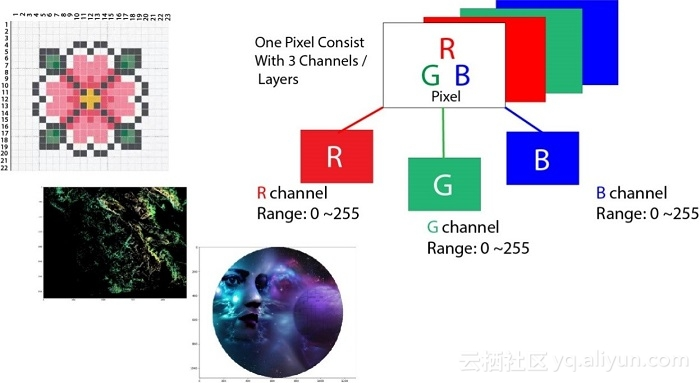对于深度学习而言，很多任务都是与数字图形处理打交道。这类任务的数据集一般是由很多张图像构成，有时候，当原始图像不能直接送入模型中时，需要对其进行一定的预处理操作，这时候就不得不向大家介绍一个十分有用的软件包OpenCV，用它处理图像起来非常方便，OpenCV是一个基于BSD许可发行的跨平台计算机视觉库，它轻量且高效，是由一系列C函数和少量C++类构成，支持Python、MATLAB等语言接口，内部包含了很多图像处理的相关算法。下面将向大家介绍如何使用NumPy和OpenCV对数字图像进行简单的处理方法：

## 关于像素的一些知识

在程序世界里，图像输入到计算机中时，与人眼所见的图像的形式不太一样。计算机将图像存储为类似于马赛克的小方块，就像古老的方块马赛克艺术的形式。如果方形块太大，那么就很难制作出光滑的边缘和曲线。使用的方块越小，则越平滑，或者说图像的像素就越少，方块的大小有时候也被称为图像的分辨率。
矢量图像是存储图像的一些不同方法，目的是为了避免与像素相关的问题。但是，即使是矢量图像，最终也会显示为像素级的马赛克。像素一词表示图像元素，描述每个像素的简单方法是使用三种颜色的组合，即红色（Red），绿色（Green），蓝色（Blue），即我们平时所说的RGB图像。

假设现在从远处观察，创建一张图像，可以看到一张图像实际上由像素点值的开关决定（像素值为1表示开，像素值为0表示关），这些开关组合创建了图像，基本上，我们每天在屏幕上看到的图像都是这种。

现在，如果RGB三个值都处于全强度，这意味着其组合值为255，该值表示为白色，如果所有三种颜色都被减弱，或者值设置为0，其值表示为黑色。反过来，三者的不同组合将为我们提供不同特定的像素颜色。由于每个数字都是8比特，因此像素值的取值范围为0-255，从下图可以看到，但R的强度为37.3%，G的强度为45.9%，B的强度为18.8%时，组合成的颜色为深绿（dark green）。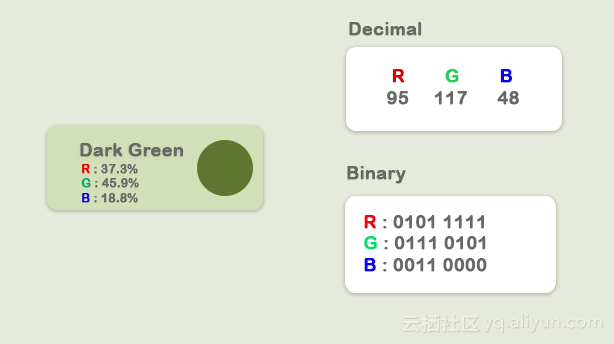三种颜色的不同组合将产生不同的颜色，由于每个值可以具有256个不同的强度或亮度值，因此总共有1680万（256 x 256 x 256）种不同组合。
图像的基本知识介绍完毕后进入正题，以下内容将包含Numpy非常基本的图像数据分析、还有一些Python数据包，比如imageio，matplotlib等。本系列博客内容结构如下，先介绍前三个部分：
• 导入图像并观察其属性
• 拆分图层
• 灰度化
• 对像素值使用逻辑运算符
• 使用逻辑运算符进行掩码
• 卫星图像数据分析

## 导入图像

下面加载图像并观察其各种属性。注意，在输入下面代码请确保好已经安装好对应的python数据包。

if__name__=='__main__':
importimageio
importmatplotlib.pyplotasplt
%matplotlibinline

plt.figure(figsize=(15,15))

plt.imshow(pic)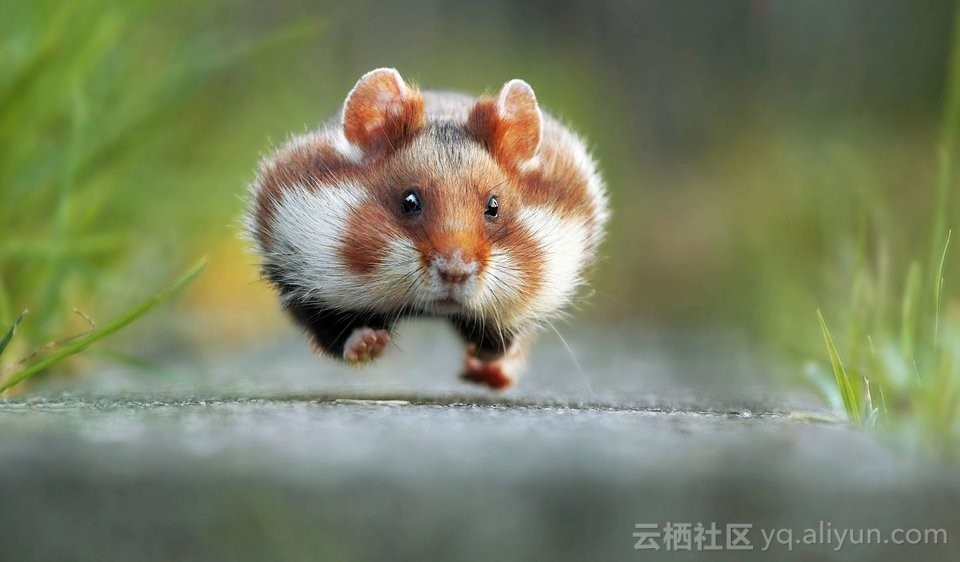## 观察图像的基本属性

rint('Type of the image : ',type(pic))
print()
print('Shape of the image : {}'.format(pic.shape))
print('Image Hight {}'.format(pic.shape))
print('Image Width {}'.format(pic.shape))
print('Dimension of Image {}'.format(pic.ndim))

Type of the image :<class 'imageio.core.util.Image'>

Shape of the image : (562, 960, 3)
Image Hight 562
Image Width 960
Dimension of Image 3

ndarray的形状表明它是一个三维矩阵，输出结果的前两个数字分别表示高度（height）和宽度（width），第三个数字（即3）表示是该图像是三通道彩色图：红色、绿色和蓝色。因此，如果我们计算RGB图像的大小，则总像素大小将是weiheigh x width x 3

print('Image size {}'.format(pic.size))

print('Maximum RGB value in this image {}'.format(pic.max()))

print('Minimum RGB value in this image {}'.format(pic.min()))

Image size 1618560

Maximum RGB value in this image 255

Minimum RGB value in this image 0

这些值对于验证而言是很重要的，因为8位颜色强度不能超出0到255范围。
使用图片可以分配变量，此外还可以访问图像的任何特定像素值，并且还可以分别访问每个RGB通道。

pic[100, 50 ]

Image([109, 143,  46], dtype=uint8)

在这种情况下：R = 109、G = 143、 B = 46，从这个配置可以看出该像素中有很多绿色，也可以通过三个通道的索引值来从中选择出一个。根据一般规定：

• 索引0表示红色通道
• 索引1表示绿色通道
• 索引2表示蓝色通道
print('Value of only R channel {}'.format(pic[ 100, 50, 0]))

print('Value of only G channel {}'.format(pic[ 100, 50, 1]))

print('Value of only B channel {}'.format(pic[ 100, 50, 2]))

Value of only R channel 109

Value of only G channel 143

Value of only B channel 46

现在快速查看整个图像中每个通道表示的图像。

plt.title('R channel')

plt.ylabel('Height {}'.format(pic.shape))

plt.xlabel('Width {}'.format(pic.shape))

plt.imshow(pic[ : , : , 0])

plt.show()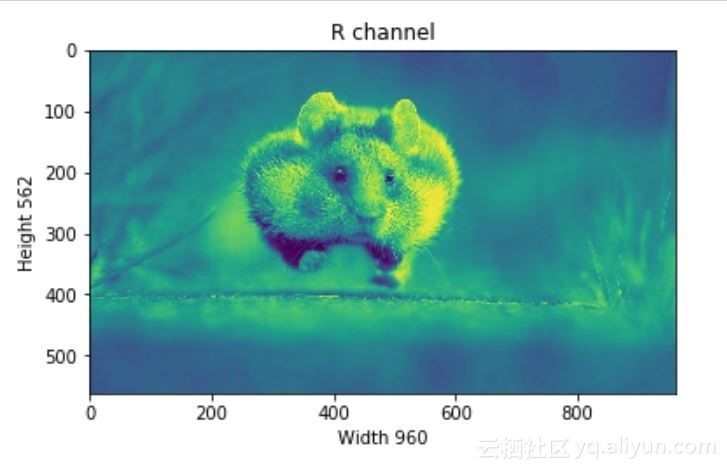plt.title('G channel')

plt.ylabel('Height {}'.format(pic.shape))

plt.xlabel('Width {}'.format(pic.shape))

plt.imshow(pic[ : , : , 1])

plt.show()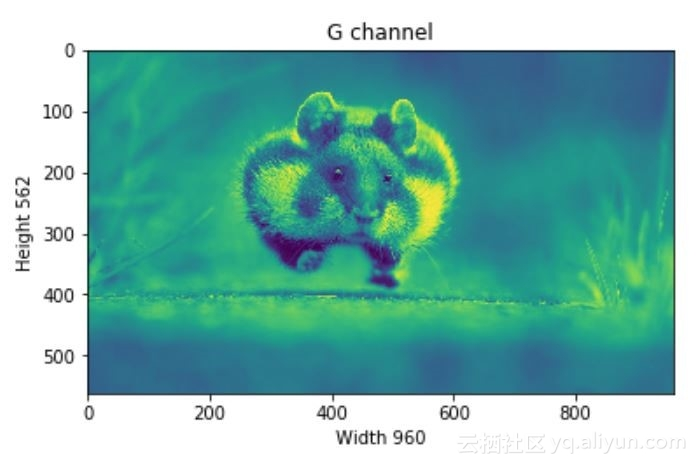plt.title('B channel')

plt.ylabel('Height {}'.format(pic.shape))

plt.xlabel('Width {}'.format(pic.shape))

plt.imshow(pic[ : , : , 2])

plt.show()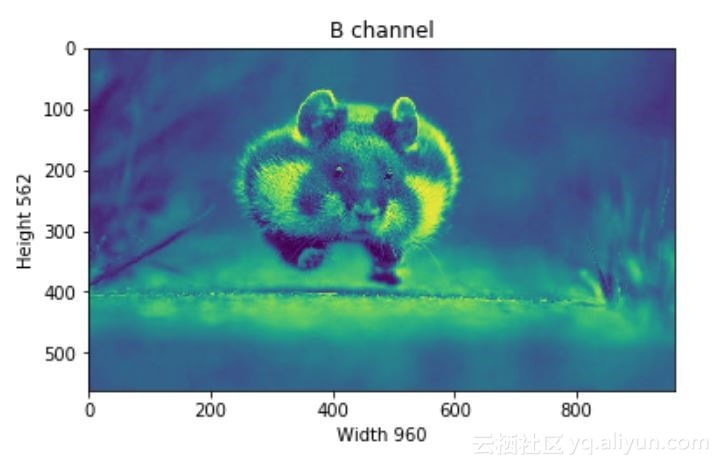下面，也可以更改RGB的数值。例如，将下面行的红色、绿色、，蓝色图层的值全部设置为全强度，即取值为255。
• R通道：第100行到110行
• G通道：第200行到210行
• B通道：行300行到310行
本次测试只在一张图像上进行综合处理，方便我们同时查看每个通道的值对图像的影响。
pic =imageio.imread('F:/demo_2.jpg')
pic[50:150 , : , 0] =255# full intensity to those pixel's R channel

plt.figure( figsize= (10,10))

plt.imshow(pic)

plt.show()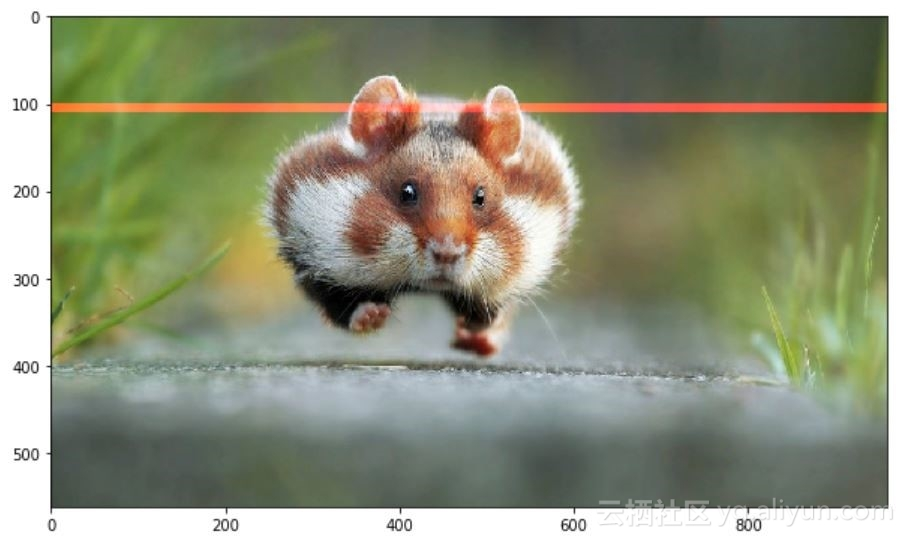pic[200:300 , : , 1] =255# full intensity to those pixel's G channel

plt.figure( figsize= (10,10))

plt.imshow(pic)

plt.show()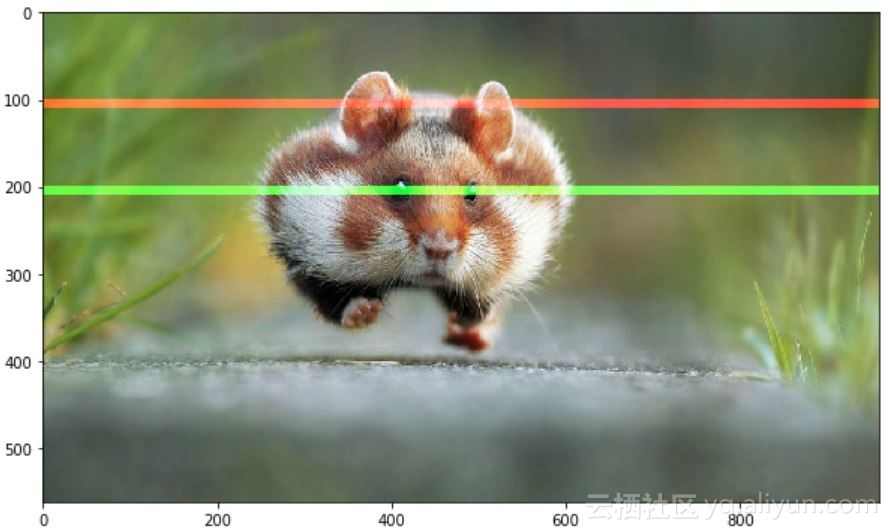pic[350:450 , : , 2] =255# full intensity to those pixel's B channel

plt.figure( figsize= (10,10))

plt.imshow(pic)

plt.show()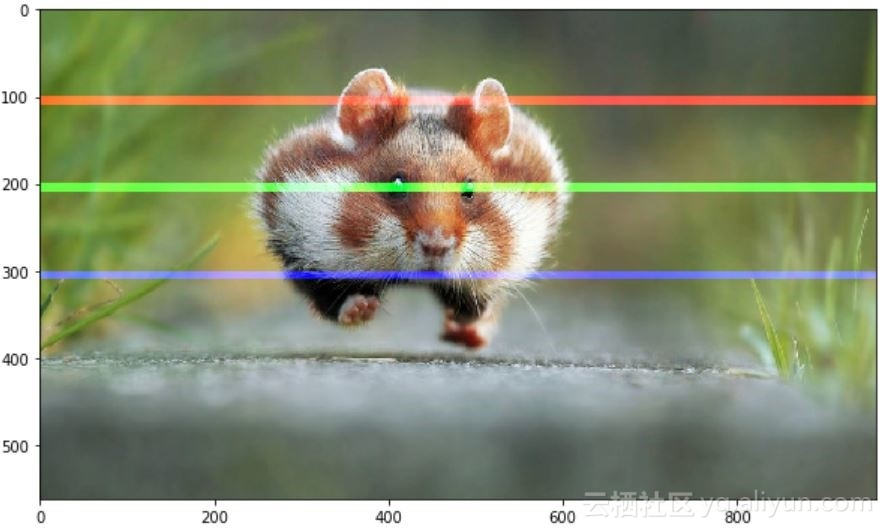为了更加清楚地对比分析，我们也改变部分列的像素值，这次测试同时更改RGB通道的值。
pic[50:450 , 400:600 , [0,1,2] ] =200

plt.figure( figsize= (10,10))

plt.imshow(pic)

plt.show()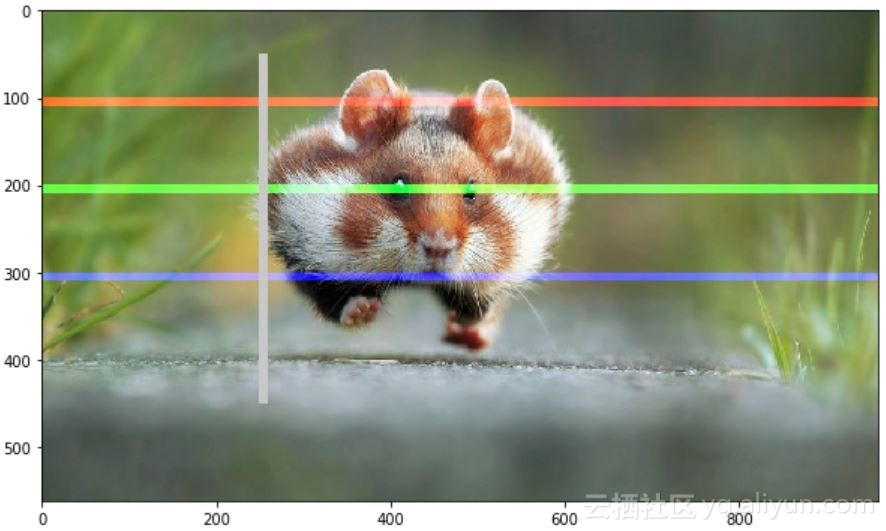## 拆分图层

通过以上测试，可以知道，图像的每个像素点都是由三个整数表示。只需要拉出图像阵列的正确切片，就可以将图像分割成单独的颜色分量。

importnumpyasnp

fig,ax=plt.subplots(nrows=1,ncols=3,figsize=(15,5))

forc,axinzip(range(3),ax):

# create zero matrix

split_img=np.zeros(pic.shape,dtype="uint8")# 'dtype' by default: 'numpy.float64'

# assing each channel split_img[:,:,c]=pic[:,:,c]

# display each channelax.imshow(split_img)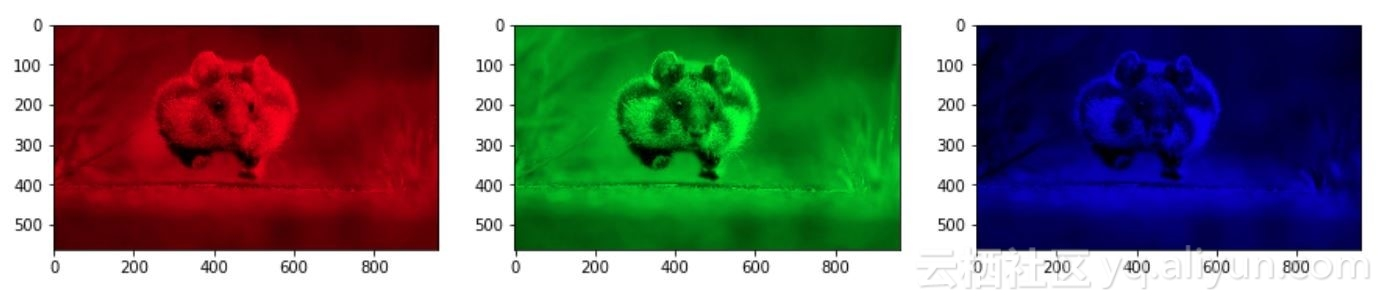## 灰度化

黑白图像存储在二维矩阵中，目前存在两种类型的黑白图像：

• 灰度：灰色阴影的范围：0~255
• 二进制：像素为黑色或白色：0或255
灰度处理过程，就是将图像从全彩色转换为灰度图。在图像处理工具中，例如：在OpenCV中，在使用很多含住之前，需要将图像进行灰度处理，这样做是因为灰度处理简化了图像，几乎像降噪一样，这是因为灰度图像中的信息比较少。

在python中有两种方法可以将图像转换为灰度。但是，更直接的方法是使用matplotlib包，该包执行的操作是获取原始图像的RGB值后进行加权平均。

Y' = 0.299 R + 0.587 G + 0.114 B
gray=lambdargb:np.dot(rgb[...,:3],[0.299,0.587,0.114])
gray=gray(pic)
plt.figure(figsize=(10,10))
plt.imshow(gray,cmap=plt.get_cmap(name='gray'))
plt.show()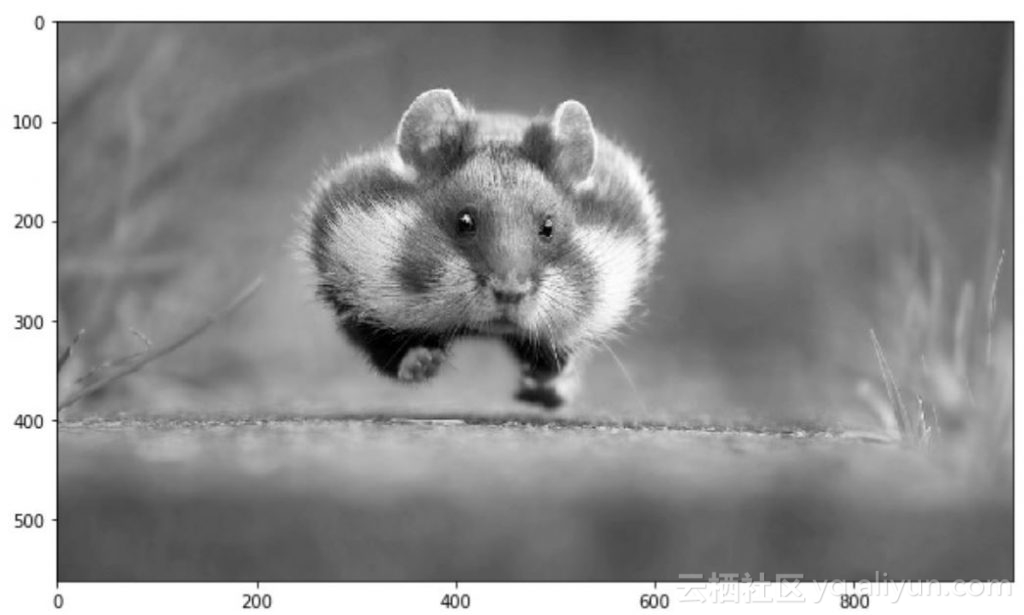而通过GIMP将颜色转换为灰度图像有三种算法来完成任务：
• 亮度（Lightness）灰度等级计算为
Lightness = ½×(max(R,G,B)+ min(R,G,B))
• 照明度(Luminosity)灰度级将计算为
Luminosity= 0.21×R + 0.7×G + 0.07×B
• 平均亮度灰度级将计算为
Average Brightness=(R + G + B)÷3

下面让我们尝试实现一下这三个算法中的一种吧，本文选择Luminosity。

pic=imageio.imread('F:/demo_2.jpg')

gray=lambdargb:np.dot(rgb[...,:3],[0.21,0.72,0.07])

gray=gray(pic)

plt.figure(figsize=(10,10))

plt.imshow(gray,cmap=plt.get_cmap(name='gray'))

plt.show()

print('Type of the image : ',type(gray))

print()

print('Shape of the image : {}'.format(gray.shape))

print('Image Hight {}'.format(gray.shape))

print('Image Width {}'.format(gray.shape))

print('Dimension of Image {}'.format(gray.ndim))

print()

print('Image size {}'.format(gray.size))

print('Maximum RGB value in this image {}'.format(gray.max()))

print('Minimum RGB value in this image {}'.format(gray.min()))

print('Random indexes [X,Y] : {}'.format(gray[100,50]))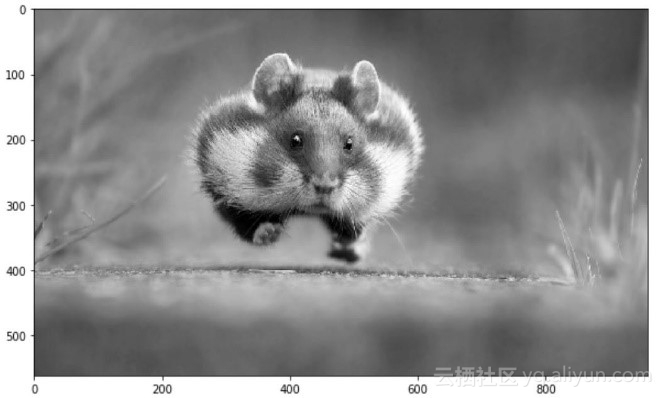Type of the image :<class 'imageio.core.util.Image'>
Shape of the image : (562,960)
Image Height 562
Image Widht 960
Dimension of Image 2
Image size 539520
Maximum RGB value in this image 254.9999999997
Minimum RGB value in this image 0.0
Random indexes [X,Y] : 129.07

## 作者信息

Mohammed Innat，机器学习和数据科学研究者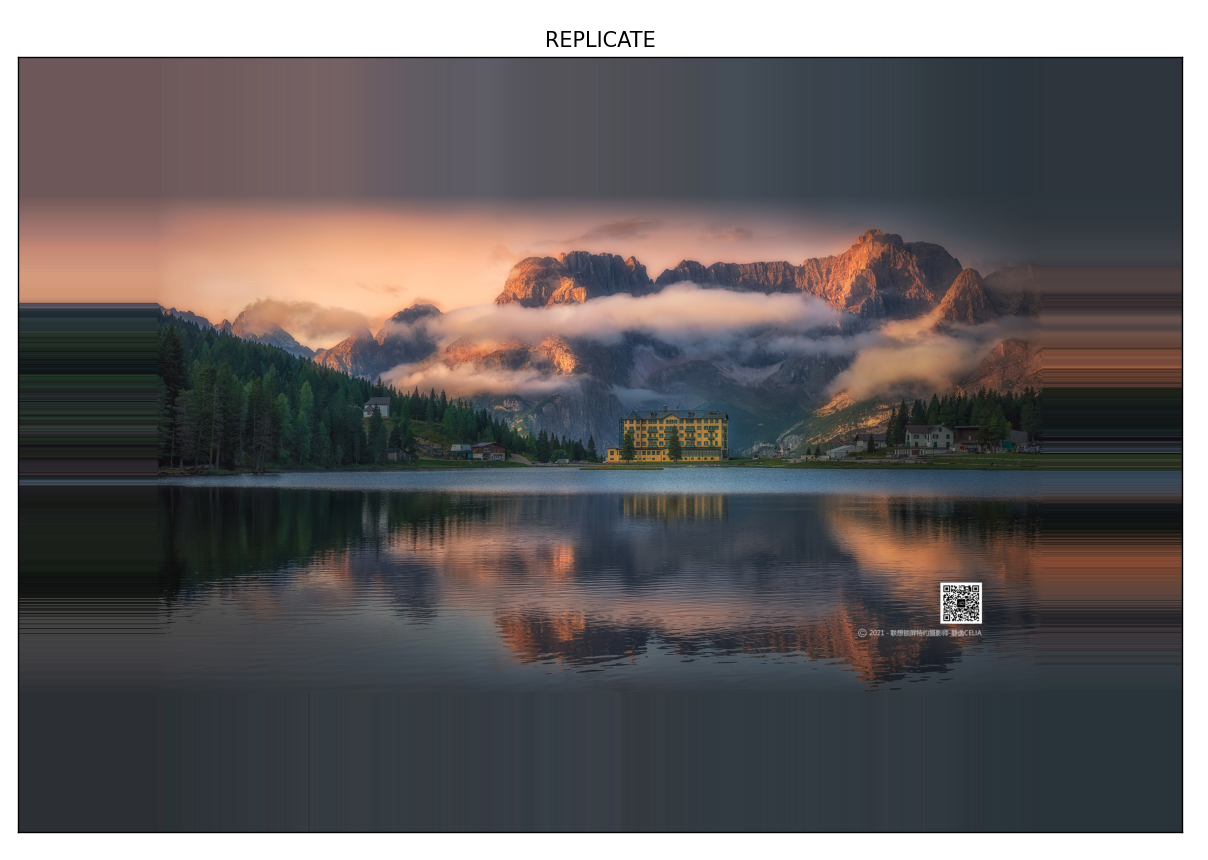CV5 numpy入门及图像的基本操作

13 0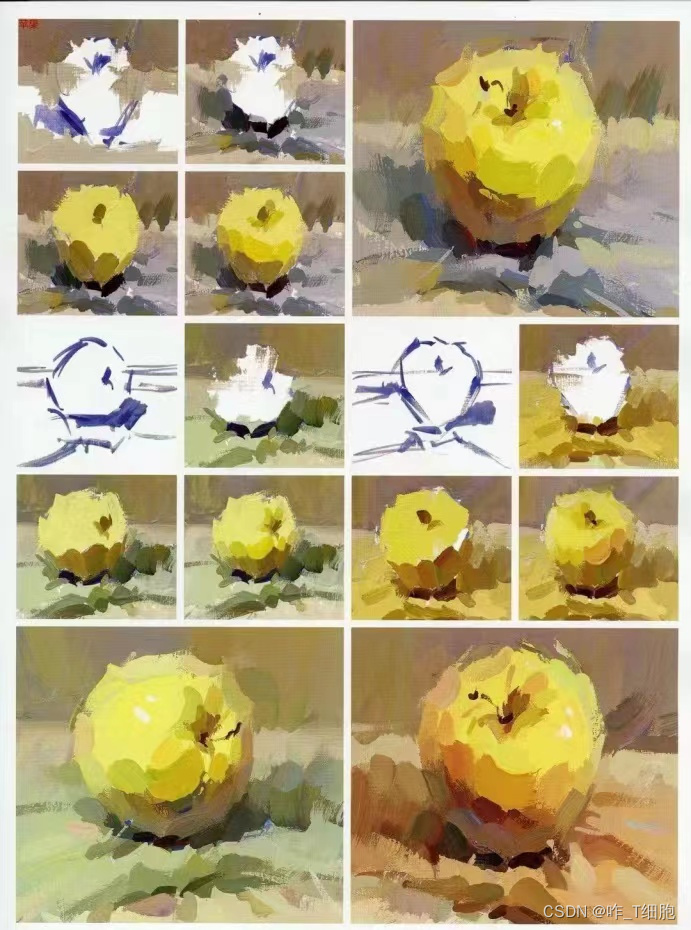93 0Python数据分析之Numpy初体验
NumPy是一个用于科学计算的Python库，主要用于快速操作数组和矩阵。NumPy提供了许多实用的函数和方法，可以大大简化科学计算的代码。
89 0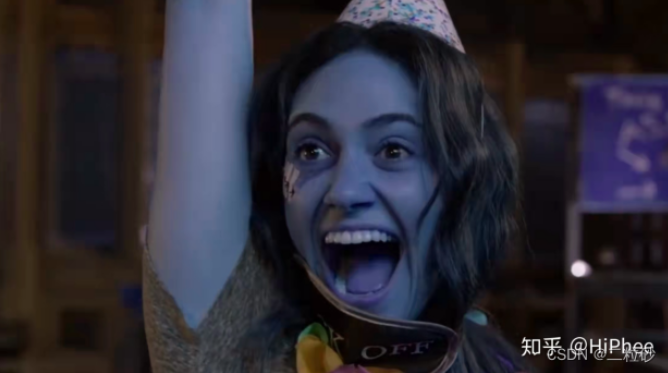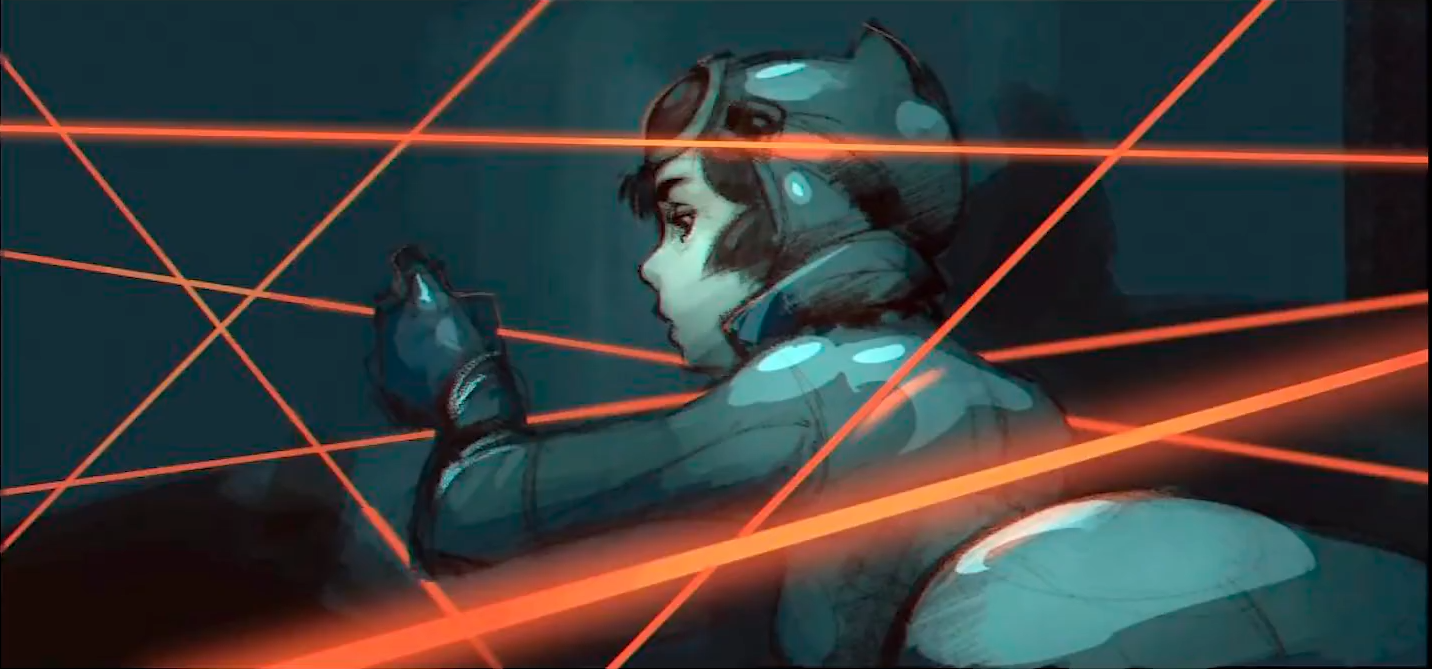【opencv】图像数据类型由numpy转为tensor后颜色改变
【opencv】图像数据类型由numpy转为tensor后颜色改变
114 0Python数据分析——numpy与pandas数据合并

60 0Python进行数据分析之科学计算库Numpy
1.遇到的一个问题 TypeError: Field elements must be 2- or 3-tuples, got ‘4‘ 错误案例：
95 0
【方向】

【视觉与图像】Python+OpenCV教程入门篇-1月16日更新9016